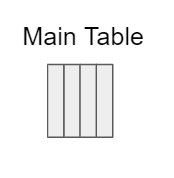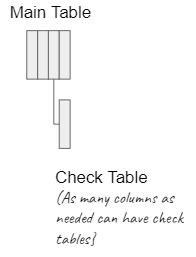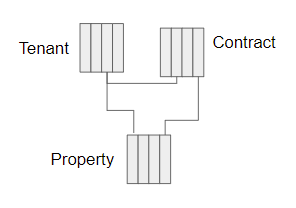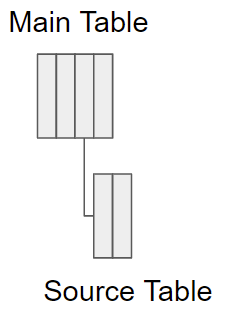Rambling Pete's Quick examples
Share
Explore#Table JoinsTable Joins## Select Lists

A select list is a type of column where you create a pre-defined check list of entries allowed in the column. It is not a Table join, since the list is not in a separate table, but it is convenient to include it in this list.
Most simple.Check tables can be implemented using the Select List column type. In this case the check values are stored in the Main Table.
Each column that is defined as a select column stores it values on the column options are.
If needed, the select values used for a select column can be moved to a standalone table.

## There are two basic ways to link tables together

For me the way to determine whether to use a Lookup column, or to build a formula using filter, is whether the tables already contain data.
If Main table is empty, and will be filled as the doc is used, then a Lookup column typically makes sense.
If you have two tables filled with data, that has a common field that you can use to join them together, then the filter() formula works well. (I often download data of sales, customers and products, which needs to be linked together to provide meaningful analysis.)
NOTE: The choice you make above, has an impact on how your data is explained in group, detail and table views. Examples are included of each view in the examples below, where applicable.
Lookup columns
Create a lookup column in your table, and link that to another table. There are some examples in the following section. Implementation range from a simple single column Lookup table, to complex multi-table, filtered lookups
Filter() in a formula column
In a formula column, create a filter to select data from the source table. Because this is a formula column, it is not possible to manually enter data into this column.
In its most simple form, you will have a one to one relationship between two tables, and filter the data from the source tables.
You can also have a complicated filter condition, using several columns, to filter data from a source table, where the filter could select more than one record. Then you need to choose do you want to see all of the values, or just the first(). Some other manipulations are also possible, like sum() or average() for numerical columns, and count() for all columns.
Examples follows below, from simple to not so simple.

### Lookup Columns

Still easy - Check tableWith a Lookup column type, it is possible to lookup a value from another table. In the Main Table, the column will provide a drop down of possible entries in the table that can be selected.
If the Check Table has more than one column, one or more of these additional columns can then also be automatically pulled into the main table.
If the Check Table has more than one column, it is possible to filter the column that is looked up from. For example, to find all the tenants in your list of business partners.

Medium to HideousIn this example we will add a 3rd table to the Lookup chain. There is no limit to the number of lookup tables that can be maintained in this way.
It is still possible in each lookup column
to pull in multiple columns from the check table
filter the lookup column

### Filter() in a formula column

Most SimpleIn this example there are two tables, one a list of people, the second a list of passport numbers. The people and passports are uniquely linked to each other via a unique key

If you are familiar with databases, you will notice that CODA does not provide the ability to create a query. However, it is possible to mimic that by creating a new table.